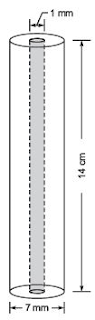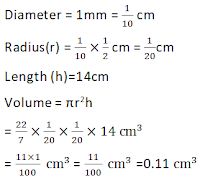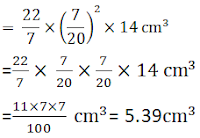#### NCERT Solutions for Class 9th: Ch 13 Surface Areas and Volumes Maths

Page No: 225

Exercise 13.4

1. Find the surface area of a sphere of radius:
(i) 10.5 cm

(ii) 5.6 cm
(iii) 14 cm

(i) Radius of the sphere (r) = 10.5 cm
Surface area = 4πr2
= (4 × 22/7 × 10.5 × 10.5) cm2
= 1386 cm2

(ii) Radius of the sphere (r) = 5.6 cm
Surface area = 4πr2
= (4 × 22/7 × 5.6 × 5.6) cm2
= 394.24 cm2

(iii) Radius of the sphere (r) = 14 cm
Surface area = 4πr2
= (4 × 22/7 × 14 × 14) cm2
= 2464 cm2

2. Find the surface area of a sphere of diameter:
(i) 14 cm
(ii) 21 cm
(iii) 3.5 m

(i) r = 14/2 cm = 7cm
Surface area = 4πr2
= (4 × 22/7 × 7 × 7)cm2
=616cm2

(ii) r = 21/2 cm = 10.5 cm
Surface area = 4πr2
= (4 × 22/7 × 10.5 × 10.5) cm2
= 1386 cm2

(iii) r = 3.5/2 m = 1.75 m
Surface area = 4πr2
= (4 × 22/7 × 1.75 × 1.75) m2
= 38.5 m2

3. Find the total surface area of a hemisphere of radius 10 cm. (Use π = 3.14)

r = 10 cm
Total surface area of hemisphere = 3πr2
= (3 × 3.14 × 10 ×10) cm2
= 942 cm2

4. The radius of a spherical balloon increases from 7cm to 14cm as air is being pumped into it. Find the ratio of surface areas of the balloon in the two cases.

Let r be the initial radius and R be the increased radius of balloons.
r = 7cm and R = 14cm
Ratio of the surface area =4πr2/4πR2
= r2/R2
= (7×7)/(14×14) = 1/4
Thus, the ratio of surface areas = 1 : 4

5. A hemispherical bowl made of brass has inner diameter 10.5 cm. Find the cost of tin-plating it on the inside at the rate of ₹16 per 100 cm2.

Radius of the bowl (r) = 10.5/2 cm = 5.25 cm
Curved surface area of the hemispherical bowl = 2πr2
= (2 × 22/7 × 5.25 × 5.25) cm2
= 173.25 cm2
Rate of tin - plating is = ₹16 per 100 cm2
Therefor, cost of 1 cm= ₹16/100
Total cost of tin-plating the hemisphere bowl = 173.25 × 16/100
= ₹27.72

6. Find the radius of a sphere whose surface area is 154 cm2.

Let r be the radius of the sphere.
Surface area = 154 cm2
⇒ 4πr2 = 154
⇒ 4 × 22/7 × r2 = 154
⇒ r2 = 154/(4 × 22/7)
⇒ r2 = 49/4
⇒ r = 7/2 = 3.5 cm

7. The diameter of the moon is approximately one fourth of the diameter of the earth. Find the ratio of their surface areas.

Let the diameter of earth be r and that of the moon will be r/4
Radius of the earth = r/2
Radius of the moon = r/8
Ratio of their surface area = 4π(r/8)2/4π(r/2)2
= (1/64)/(1/4)
= 4/64 = 1/16
Thus, the ratio of their surface areas is 1:16

8. A hemispherical bowl is made of steel, 0.25 cm thick. The inner radius of the bowl is 5 cm. Find the outer curved surface area of the bowl.

Inner radius of the bowl (r) = 5 cm
Thickness of the steel = 0.25 cm
∴ outer radius (R) = (r + 0.25) cm
= (5 + 0.25) cm  = 5.25 cm
Outer curved surface = 2πR2
= (2 × 22/7 × 5.25 × 5.25) cm2
= 173.25 cm2

9. A right circular cylinder just encloses a sphere of radius r (see Fig. 13.22). Find
(i) surface area of the sphere,
(ii) curved surface area of the cylinder,
(iii) ratio of the areas obtained in (i) and (ii).(i) The surface area of the sphere with raius r = 4πr2

(ii) The right circular cylinder just encloses a sphere of radius r.
∴ the radius of the cylinder = r and its height = 2r
∴ Curved surface of cylinder =2πrh
= 2π × r × 2r
= 4πr2
(iii) Ratio of the areas = 4πr2:4πr2 = 1:1

Page No: 228

Exercise 13.5

1. A matchbox measures 4cm × 2.5cm × 1.5cm. What will be the volume of a packet containing 12 such boxes?

Dimension of matchbox = 4cm × 2.5cm × 1.5cm
l = 4 cm, b = 2.5 cm and h = 1.5 cm
Volume of one matchbox = (l × b × h)
= (4 × 2.5 × 1.5)  cm3 = 15 cm3
Volume of a packet containing 12 such boxes = (12 × 15)  cm3 = 180 cm3

2. A cuboidal water tank is 6 m long, 5 m wide and 4.5 m deep. How many litres of water can it hold? (1m3 = 1000 l)

Dimensions of water tank = 6m × 5m × 4.5m
l = 6m , b = 5m and h = 4.5m
Therefore Volume of the tank =ℓbh m3
=(6×5×4.5)m3=135 m3
Therefore , the tank can hold = 135 × 1000 litres  [Since 1m3=1000litres]
= 135000 litres of water.

3. A cuboidal vessel is 10 m long and 8 m wide. How high must it be made to hold 380 cubic metres of a liquid?

Length = 10 m , Breadth = 8 m and Volume = 380 m3
Volume of cuboid = Length × Breadth × Height
⇒ Height = Volume of cuboid/(Length × Breadth)
= 380/(10×8) m
= 4.75m

4. Find the cost of digging a cuboidal pit 8 m long, 6 m broad and 3 m deep at the rate of ₹30 per m3.

l = 8 m, b = 6 m and h = 3 m
Volume of the pit = lbh m3
= (8×6×3) m3
= 144 m3
Rate of digging = ₹30 per m3
Total cost of digging the pit = ₹(144 × 30) = ₹4320

5. The capacity of a cuboidal tank is 50000 litres of water. Find the breadth of the tank, if its length and depth are respectively 2.5 m and 10 m.

length = 2.5 m, depth = 10 m and volume = 50000 litres
1m3 = 1000 litres

∴ 50000 litres = 50000/1000 m3 = 50 m3
= 50/(2.5×10) m
= 2 m

6. A village, having a population of 4000, requires 150 litres of water per head per day. It has a tank measuring 20m × 15m × 6m. For how many days will the water of this tank last?

Dimension of tank = 20m × 15m × 6m
l = 20 m , b = 15 m and h = 6 m
Capacity of the tank = lbh m3
= (20×15×6) m3
= 1800 m3
Water requirement per person per day =150 litres
Water required for 4000 person per day = (4000×150) l
= (4000×150)/1000
= 600 m3
Number of days the water will last = Capacity of tank Total water required per day
=(1800/600) = 3
The water will last for 3 days.

7. A godown measures 40m × 25m × 15m. Find the maximum number of wooden crates each measuring 1.5m × 1.25m × 0.5m that can be stored in the godown.

Dimension of godown = 40 m × 25 m × 15 m
Volume of the godown = (40 × 25 × 15) m3 = 10000 m3
Dimension of crates = 1.5m × 1.25m × 0.5m
Volume of 1 crates = (1.5 × 1.25 × 0.5) m3 = 0.9375 m3
Number of crates that can be stored =Volume of the godown/Volume of 1 crate
= 10000/0.9375 = 10666.66 = 10666

8. A solid cube of side 12 cm is cut into eight cubes of equal volume. What will be the side of the new cube? Also, find the ratio between their surface areas.

Edge of the cube = 12 cm.
Volume of the cube = (edge)3 cm3
= (12 × 12 × 12) cm3
= 1728 cm3
Number of smaller cube = 8
Volume of the 1 smaller cube =1728/8 cm3 = 216 cm3
Side of the smaller cube = a
a= 216
⇒ a = 6 cm
Surface area of the cube = 6 (side)2
Ratio of their surface area = (6 × 12 × 12)/(6 × 6 × 6)
= 4/1 = 4:1

9. A river 3 m deep and 40 m wide is flowing at the rate of 2 km per hour. How much water will fall into the sea in a minute?

Depth of river (h) = 3 m
Width of river (b) = 40 m
Rate of flow of water (l) = 2 km per hour = (2000/60) m per minute
= 100/3 m per minute
Volume of water flowing into the sea in a minute = lbh m3
= (100/3 × 40 × 3) m3
= 4000 m3

Page No: 230

Exercise 13.6

1. The circumference of the base of a cylindrical vessel is 132 cm and its height is 25 cm. How many litres of water can it hold? (1000 cm 3 = 1l)

Let the base radius of the cylindrical vessel be ‘r’ cm.
∴ Circumference = 2πr
⇒ 2πr = 132     [ Circumference = 132 cm]
⇒ 2× 22/7 × r = 132 cm
R=(132×7)/(2×22) cm = 21 cm
∵ Height of the vessel = 25 cm
∴ Volume = πr2 x h    [ Volume of a cylinder = πr2h]
= (22/7) (21)2 × 25 cm3
= (22/7) × 21 × 21 × 25 cm3
= 22 × 3 × 21 × 25 cm3
= 34650 cm
∵ Capacity of the vessel = Volume of the vessel
∴ Capacity of cylindrical vessel = 34650 cm
Since 1000 cm= 1 litre
⇒ 34650 cm3 = (34650/1000) litres = 34.65 l

2. The inner diameter of a cylindrical wooden pipe is 24 cm and its outer diameter is 28 cm. The length of the pipe is 35 cm. Find the mass of the pipe, if 1 cm3 of wood has a mass of 0.6 g.

Here, Inner diameter of the cylindrical pipe = 24 cm
⇒ Inner radius of the pipe (r) = (24/2) cm = 12 cm
Outer diameter of the pipe = 28 cm
⇒ Outer radius of the pipe (R) = (28/2)cm = 14 cm
Length of the pipe (h) = 35 cm
∵ Inner volume of the pipe = πr2h
Outer volume of the pipe = πr2h
∴ Amount of wood (volume) in the pipe = Outer volume – Inner volume
= πR2h - πr2h
= πh(R2-r2)
= πh(R+r)(R-r) [ a2 - b2 = (a+b)(a-b)]
= 22/7 x 35 x (14+12) x (14-12) cm3
= 22 x 5 x 26 x 2 cm3

Mass of the wood in the pipe = [Mass of wood in 1 m3 of wood] x [Volume of wood in the pipe]
= [0.6g] x [22 x 5 x 26 x 2] cm3
= (6/10)x 22 x 10 x 26 g = 6 x 22 x 26 g
= 3432 g = (3432/1000)= 3.432 kg   [ 1000 g = 1 kg]
Thus, the required mass of the pipe is 3.432 kg.

3. A soft drink is available in two packs (i) a tin can with a rectangular base of length 5 cm and width 4 cm, having a height of 15 cm and (ii) a plastic cylinder with circular base of diameter 7 cm and height 10 cm. Which container has greater capacity and by how much?

For rectangular pack: Length (l) = 5 cm
Breadth (b) = 4 cm Height (h) = 15 cm
∴ Volume = l x b x h = 5 x 4 x 15 cm3
= 300 cm3
⇒ Capacity of the rectangular pack = 300 cm3     ...(1)
For cylindrical pack: Base diameter = 7 cm
⇒ Radius of the base (r) = (7/2)cm
Height (h) = 10 cm
∴ Volume = πr2h = (22/7) x (7/2)2 x 10 cm3
= (22/7) x (7/2) x (7/2) x 10 cm3
= 11 x 7 x 5 cm3 = 385 cm3
⇒ Volume of the cylindrical pack = 385 cm3   ...(2)
From (1) and (2),
we have 385 cm3 – 300 cm3 = 85 cm3
⇒ The cylindrical pack has the greater capacity by 85 cm3.

4. If the lateral surface of a cylinder is 94.2 cmand its height is 5 cm, then find: (i) radius of its base (ii) its volume. (Use π = 3.14)

(i) Since lateral surface of the cylinder = 2 πrh
But lateral surface of the cylinder = 94.2 cm2
2πrh = 94.2
2×3.14×r×5 =942/10
⇒{(10×314)/100} × r = 942/10
⇒ r=(942/10)×{100/(10×314)}cm
⇒ r =471/157 cm
Thus, the radius of the cylinder = 3 cm

(ii) Volume of a cylinder = πr2h
⇒ Volume of the given cylinder = 3.14 x (3)2 x 5 cm3
=314×100×3×3×5cm3
=(157×3×3)/10
= 1413/10 = 141.3 cm3

Thus, the required volume = 141.3 cm3

5. It costs ₹ 2200 to paint the inner curved surface of cylindrical vessel 10 m deep. If the cost of painting is at the rate of ₹ 20 per m2; find: (i) inner curved surface of the vessel (ii) radius of the base (iii) capacity of the vessel.

(i) To find inner curved surface
Total cost of painting = ₹ 2200
Rate of painting = ₹ 20 per m2
∴  Area =cost/rate = 2200/20 = 110 m2
⇒ Inner curved surface of the vessel = 110 m2

(ii) To find radius of the base Let the base radius of the cylindrical vessel.
∵ Curved surface of a cylinder = 2 πrh
∴  2πrh = 110
⇒ 2× 22/7 ×r×10 = 110 [∵Height=10 m ]
⇒ r= (110×7)/(2×22×10)m = 7/4 m
= 1.75 m
⇒ The required radius of the base = 1.75 m

(iii) To find the capacity of the vessel
Since, volume of a cylinder = πr2h
∴ Volume (capacity) of the vessel =22/7 × (7/2)2 × 10 m3
= 22/7 × 7/4 × 7/4 × 10 m3
= (11×7×5)/4 m3 = 385/4 m3 = 96.25 m3

Since, 1 m3 = 1000000 cm3 = 1000 l = 1 kl
∴ 96.5 m3 = 96.5 kl
Thus, the required volume = 96.25 kl

6. The capacity of closed cylindrical vessel of height 1 m is 15.4 litres. How many square metres of metal sheet would be needed to make it?

Capacity of the cylindrical vessel = 15.4 l
= 15.4×1000 cm⇒ Volume of the vessel = (15.4/1000)m3
Height of the vessel = 1m Let ‘r’ metres be the radius of the base of the vessel
∴ Volume = πr2h
⇒ πr2h= 15.4/1000Thus, the required sheet = 0.4708 m2

7. A lead pencil consists of a cylinder of wood with a solid cylinder of graphite filled in the interior. The diameter of the pencil is 7 mm and the diameter of graphite is 1 mm. If the length of the pencil is 14 cm, find the volume of the wood and that of the graphite.

Since, 10 mm = 1cm
∴ 1 mm = (1/10) cm
For graphite cylinderThus, the required volume of the graphite = 0.11 cm
For the pencil Diameter of the pencil = 7 mm = (7/10)cm
∴ Radius of the pencil (R) = (7/20) cm
Height of the pencil (h) = 14 cm
Volume of the pencil = πr2hVolume of the wood Volume of the wood = [Volume of the pencil] – [Volume of the graphite]
= 5.39 cm3 – 0.11 cm3 = 5.28 cm
Thus, the required volume of the wood is 5.28 cm3.

8. A patient in a hospital is given soup daily in a cylindrical bowl of diameter 7 cm. If the bowl is filled with soup to a height of 4 cm, how much soup the hospital has to prepare daily to serve 250 patients?

The bowl is cylindrical.
Diameter of the base = 7 cm
⇒ Radius of the base (r) = (7/3) cm
Height (h) = 4 cm
Volume of soup = πr2h= 38500 / 100 liters
Thus, the hospital needs to prepare 38.5 litres of soup daily for 250 patients.

Go to Part I (Exercise 13.1 to 13.3)

Go to Part III (Exercise 13.7 to 13.8)

Go To Chapters